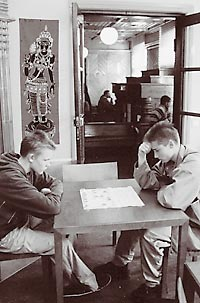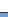# A short introduction to the most common xiangqi openings

Some of the most important xiangqi openings are introduced here. The purpose is to help new players to get started with the game. This kind of short introduction can naturally not be very comprehensive. It only contains few examples of the most common opening variations. Some openings have been introduced with only one line. Our source of information has been Chinese opening books. We have used the Chinese notation system and the names and the abbreviations for the pieces follow the WXF (World Xiangqi Federation) standard. (K=King, R=Chariot, H=Horse, C=Cannon, E=Elephant, A=Advisor, P=Pawn) The names for the openings follow the practice of English xiangqi books. In some cases the Chinese names have also been mentioned.

The xiangqi openings follow the same principles as the chess openings. There are naturally also some differences. The most important thing in the opening is the quick development of the pieces. Moving the center pawn (P5+1) in the opening, which is common in chess, is a mistake in xiangqi. After the reply C2=5 (or C8=5) red loses his center pawn! The center line is strategically important but moving the center pawn makes sense only later when the player is ready to attack. Moving the 3. or 7. line (c and g line in algebraic notation) pawn is a useful development move because after that the horse can advance to the center and the pawn move hinders the corresponding opponent move. The important question is how to arrange the horses, cannons and elephants on the 3. rank. The chariots should be developed as soon as possible.

The most popular and aggressive opening move is 1.C2=5. It has been estimated that about 70% of all games begin with this move. The cannon threatens the opponents center pawn and it prepares for the attack. Attempting to win material and neglecting the development with moves like 1.C2=3 can be a dangerous waste of time.

Black has four very popular and reliable defences against the central cannon opening.

”Screen horses” aka “Two horses defence” (Ping Feng Ma) is the most popular black defence. Black moves both of his horses between the cannons to protect the center pawn. This opening has several variations.

”Fan Gong Ma” (The name is difficult to translate) is another opening where black developes both his horses towards the center but one of the cannon is paced on the palace corner between the horses. black can now connect his elephants. This opening is relatively new. Red has several ways to attack but the black position is solid.

”Same direction Cannon” opening starts with the moves 1.C2=5, C8=5. Black replies with a symmetrical move. This cannon move is possible because the immediate capture of the defenceless center pawn with 2.C5+4 loses time and black gets the initiative. This capture is only played by amateurs.

”Opposite direction Cannon” opening starts with the moves 1.C2=5, C2=5. Here red and black move their cannons to the opposite directions. The modern practice is to make the black cannon move later. (“Delayed opposite direction Cannon”).

The fifth interesting alternative is ”The Three Step Tiger” opening. This name refers to the system where the player develops his chariot quickly by moving the cannon to the edge of the board. (For example with the moves H2+3, C2=1 and R1=2)  This way of development can lead to other openings

Other defences against the central cannon are rare.

Red naturally has other opening moves besides the central cannon. These systems are sometimes called “Soft openings” because the opening move is not a threat.

”The pawn opening” (1.P7+1) is a very flexible system. The red opening move is a useful development move but all the choises concerning piece development are still open. Black usually does not answer with the central cannon because red could then play any of the central cannon openings with colors reversed and the pawn move would be an extra advantage.

”The elephant opening” (1.E3+5) is a very solid opening where red first protects his King by connecting the elephants.

”The horse opening” (1.H2+3) starts with a natural development move. Red can play the Two horse defence, The Fan Gong Ma or Three step tiger opening with the colors reversed.

Red can also play his cannon to the front corner of the palace with the move 1.C2=4 (”Palcorner Cannon”) or 1.C2=6 (“Crosspalace Cannon”). These moves are also useful developing moves.

Other red opening moves like 1.H8+9 (”Edge Horse”), 1.C2=3 or 1.C2=7 are very rare.

Chess grandmaster Robert Hubner, who has also played Chinese chess, has said that the Chinese chess does not have as much opening theory as chess but there is more endgame theory.

Olavi Stenman and Timo Aitta

THE MOST COMMON XIANGQI OPENINGS (17.1.2007 / TA,OS)

1) Central Cannon Openings

Two horse defence, Screen horses   1. C2=5 H8+7  2. H2+3 R9=8

3. P7+1 P7+1  4. H8+7 H2+3  5. R1+1 /C8+2 /C8=9

3. R1=2 P7+1  4. P7+1 C8+4  5. H8+7 E3+5 /C2=5

4. R2+6 H2+3  5. P5+1 A4+5

5. C8=6 R1=2  6. H8+7 C2=1  7. P5+1

5. H8+7 P3+1  6. R9+1 C2+1  7. R2-2 E3+5  8. P3+1 /P7+1 /R9=6   (“Two headed

H2+3  4. P3+1 P3+1  5. C8+4 E7+5 /H3+2                                                                               snake“)

5. H8+9 E3+5  6. C8+4

E7+5  6. R9+1

P1+1  6. C8=7 H3+2  7. R9+1 P1+1 /E7+5 /E3+5

4. H8+9 P7+1  5. C8=6 R1=2  6. R9=8 C2+4  7. R2+4 C8=9  8. R2=4

5. C8=7 R1=2  6. R9=8 C2+4 / C2+2 /C8+4

4. P7+1 P7+1  5. C8=7 C8+2  6. H8+9

C2+4  6. R2+4

5. H8+7 E3+5  6. C8+2

C8+2  6. H7+6

C2+4  6. R2+4 C2=7  7. E3+1

6. P5+1 C8+4                    (“River crossed cannons”)

5. R2+6 R1+1 6. H8+7 /C8=7

H7+6  6. H8+7 E3+5/R1+1/P7+1

C2+4  6. P5+1 E3+5  7.H8+7 A4+5  8. R9+1/C8=9

A4+5  6. H8+7 /C8=7

C8=9  6. R2=3 R8+2  7. H8+7 E3+5                                   (“Edge cannon”)

C9-1  7. P5+1 /H8+9/C8=6

7. H8+7 R1+1  8. C8=9

A4+5  8. H7+6/C8=9

Delayed opposite direction cannon   1. C2=5 H8+7  2. H2+3 R9=8  3. R1=2

C2=5  4. R2+6 /H8+7

C8+4  4. P3+1 C2=5  5. C8+5 H2+3                                                              (“Modern variation”)

5. H8+7 R1+1  6. R9=8 R1=8  7. H3+4 /P7+1

H2+3  6. P7+1 R1=2  7. R9=8 R2+6  8. H7+6 /C8=9

R2+4  8. C8=9 R2=8  9. R8+6 P7+1/C8=7/C5=6

6. R9=8 P3+1  7. H3+4 C8+1/R1=2/R1+1

7. C8+4 C8=7  8. C8=7

Parrot cannon    1. C2=5 H8+7  2. H2+3 R9+1  3.R1=2 C8-1  4. H8+9 E3+5

4. H8+7 P3+1

Three step tiger (Left)   1. C2=5 H8+7  2. H2+3 R9=8  3. P7+1 C8=9

4. H8+7 C2=5  5. R9=8 H2+3  7. P3+1/R1+1

P7+1  5. R1+1/C8+2 /H7+6

R8+5  5. P5+1 /E7+9

Three step tiger (Left)  1. C2=5 H2+3  2. H2+3 T2=1  3. H8+7 R1=2  4. R9=8 R2+6  5. C8=9

Fan Gong Ma   1.C2=5  H2+3  2. H2+3 C8=6

3. R1+1 H8+7  4. R1=4 R9=8  5. H8+7 A4+5  6. P5+1/R9+1

3. R1=2 H8+7  4. C8=6 R1=2  5. H8+7 C2=1  6. P7+1 P7+1  7. H7+6 A6+5

4. P7+1 P7+1  5. R2+6 R9+2/A4+5

4. P3+1 P3+1  5. H8+9 E3+5  6. C8=6/C8=7

E7+5  6. C8=6/C8=7

One horse defence    1. C2=5 H2+3  2. H2+3 R9+1  3. R1=2 H8+9  4. P7+1 C8=7  5. H8+7 E3+5 /R9=4

Yuan Yang cannons  1. C2=5 H2+3  2. H2+3 P7+1  3. R1=2 R9+2  4. H8+7 C2-1  5. R2+6

Same direction cannon     1. C2=5 C8=5  2. H2+3 H8+7

3. P3+1 R9=8/R9+1

3. R1+1 R9=8  4. R1=6 R8+4 5. H8+7 H2+3/A6+5

3. R1=2 P7+1  4. H8+7 H2+3  5. P7+1 R1+1

4. P7+1 C2+4  5. H8+7 H2+3/R9+1

R9+1  5. H8+7 R9=4  6. R2+4 H2+3  7. P3+1

R9+1 4. R2+6 P3+1  5. R2=3 H2+3

5. C8=7 H2+3  6. P7+1 H3+4

4. C8=6 H2+3  5. H8+7 R1=2  6. R9=8 C2+4  7. C6+5 R9=7  8. R2+6 H7-9

R9=4  5. A4+5 H2+1  6. H8+7 R1=2  7. R9=8 R4+5  8. R2+6 C5=3

4. H8+7 H2+3  5. P7+1 R1+1  6. P3+1/C8+1

R9=4  5. P7+1 H2+1 /H2+3/R4+5

5. P3+1 R4+4  6. C5=4 /E3+1

P3+1  6. R2+5 E3+1  7. C8=9

H2+1  6. H3+4

H2+3  6. P7+1 R1+1  7. A6+5 R4+5  8. E7+9   (“Double head snake”)

Opposite direction cannon   1. C2=5 C2=5  2. H2+3

H2+3  3. R1=2 R1=2  4. H8+7 H8+7  5. R9=8 R9=8  6. C8+4

H8+7  4. C8=6

H8+9  3. R1=2 R9=8  4. H8+9 /H8+7

2) Other Openings

Pawn opening    1.P7+1

P7+1            2. C2=3 C8=5

2. H8+7 H8+7  3. R9+1/C8=9

H8+7            2. P3+1 C2=3/C8=9/R9+1

C2=3            2. C2=5 E3+5  3. A6+5 P7+1/H8+7

3. H8+9 R9+1/H8+7

3. H2+3 R9+1  4. R1=2 R9=2  5. H8+7 H2+4  6. C8=9 H8+9  7. H7+6

P3+1  4. R1=2 P3+1  5. H8+9     (“Gambit variaton”)

Elephant opening    1. E3+5  P7+1  2. H8+9/P7+1

C8=5  2. H2+3 H8+7  3. R1=2 R9=8  4. H8+7 H2+1/R8+6/P7+1

C8=4  2. H2+3 H8+7  3. R1=2 H2+1/P7+1

C2=4  2. R9+1 H2+3  3. R9=6 H8+7  4. H8+9 R1=2  5. P9+1

Horse opening     1. H2+3 P7+1  2. P7+1 H8+7  3. H8+7 R9+1/H2+3

2. C2=1 H8+7  3. R1=2 R9=8  4. R2+6 C8=9

2. C8=6 H8+7  3. H8+7 H2+1

Palcorner cannon   1. C2=4 C2=5  2. H8+7 H2+3  3. H2+3 H8+9  4. R1=2 R9=8  5. R9=8 R1=2  6. C4+5/C8+4

Crosspalace cannon   1. C2=6 C8=5  2. H2+3 H8+7  3. R1=2 R9+1  4. R2+6 R9=4  5. A4+5 H2+3Main Page

Discussion Forum